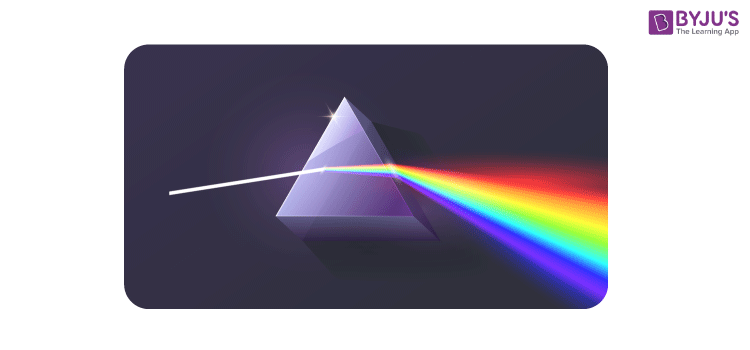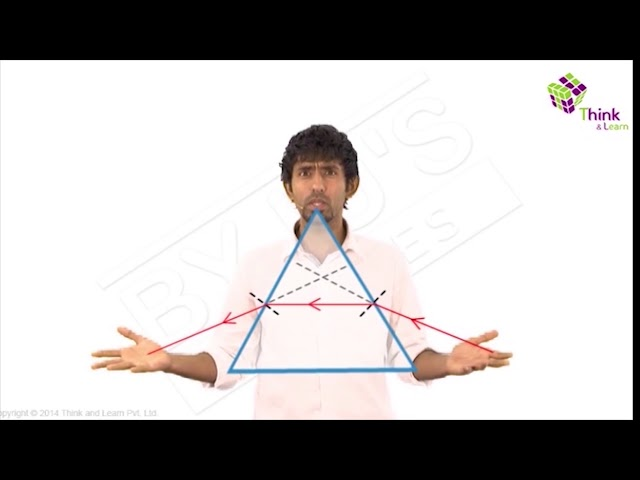# Glass Prism - Dispersion Of White Light

## What is Prism?

In optics, a prism is defined as a transparent solid body that has three rectangular lateral surfaces and two triangular faces that are inclined at an angle. The angle at which the surfaces are inclined is known as the angle of the prism. It is also known as refracting angle. The other important term in the prism is the angle of deviation of a prism. It is defined as the angle between the incident ray and the emergent ray. Following is the formula used for calculating the angle of deviation:

 i1 + i2 = A + δ

Learn how to trace the path of the rays using glass prism here.

## What is Dispersion?

Dispersion is defined as the separation of white light into different colors when the light is passed through the prism. The scattering of light depends on the wavelength of the light. Therefore, it can be said that the degrees of deviation are dependent on the wavelengths. The deviation in the path of the light is inversely proportional to the wavelength.

White light is primarily composed of light of different wavelengths (colors) viz. violet, indigo, blue, green, yellow, and red with red having the highest wavelength while the violet having the lowest wavelength.

Red light suffers the least amount of deviation and violet the most. Since all the wavelengths suffer different angles of deviation, when white light passes from one optical medium to another, different colors of the light split, and this phenomenon of splitting of light into its components as a result refraction is called dispersion.## Dispersion of White Light by a Glass Prism

When white light is made to pass through a prism, a spectrum of seven colors is formed which shows that white light is a mixture of seven different colors. Prism only acts as a medium for the separation of the seven colors. When light falls on the glass prism, refraction takes place. Since the wavelength of different components of light is different and the frequency is constant, each component gets deviated by a different angle due to the difference in velocity in the glass medium. The red color having the maximum wavelength deviates the least and forms the upper part of the spectrum whereas violet having the least wavelength deviates the most.Learn more about different phenomena associated with light waves and their behaviour under different conditions with the team of expert mentors at BYJU’S.

Test your knowledge on Dispersion in prism